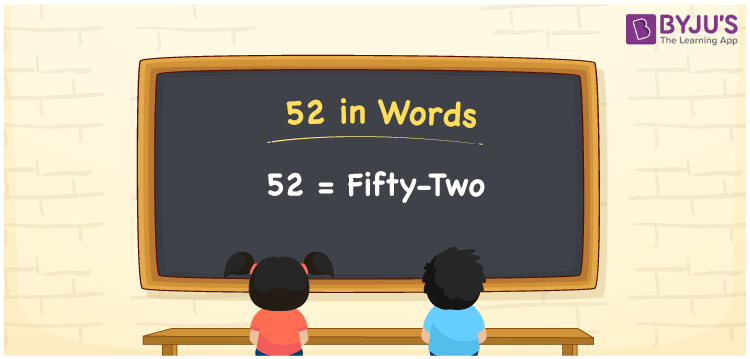# 52 in Words

52 in words can be written as Fifty-Two. This article will provide you with the complete idea of how numbers can be written into words with the help of the English alphabet. If you buy 1 dozen eggs for Rs. 52, then you can say that “I bought 1 dozen eggs for Fifty-Two Rupees”. Learn more about the numbers in words concept using the reference materials provided at BYJU’S. 52 can be read as “Fifty-Two” in English.

 52 in words Fifty-two Fifty-two in Numbers 52

## 52 in English Words## How to Write 52 in Words?

Learn in detail about the place value chart of 52 here. There are two digits in 52 and students can learn more about it from the table shown below.

 Tens Ones 5 2

52 in expanded form can be written as:

5 × Ten + 2 × One

= 5 × 10 + 2 × 1

= 50 + 2

= 52

= Fifty-two

Hence, 52 in words is written as Fifty-two.

52 is a natural number that precedes 53 and succeeds 51.

52 in words – Fifty-two

Is 52 an odd number? – No

Is 52 an even number? – Yes

Is 52 a perfect square number? – No

Is 52 a perfect cube number? – No

Is 52 a prime number? – No

Is 52 a composite number? – Yes

## Frequently Asked Questions on 52 in Words

Q1

### Write 52 in words.

52 can be written in words as “Fifty-two”.
Q2

### How to write Fifty-two in numbers?

Fifty-two can be written in numbers as 52.
Q3

### Is 52 a perfect cube number?

No, 52 is not a perfect cube number as it cannot be shown as a product of three same numbers.